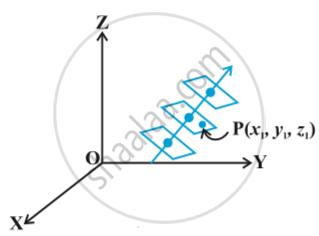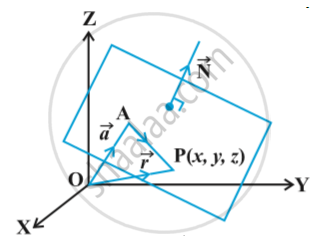# Plane - Equation of a Plane Perpendicular to a Given Vector and Passing Through a Given Point

## Notes

There can be many planes that are perpendicular to the given vector, but through a given point P(x_1, y_1, z_1), only one such plane exists in following fig.Let a plane pass  through a point A with position vector vec a and perpendicular to the vector
vec N
Let vec r be the position vector of any point P(x,y,z) in the plane. Fig.Then the point P lies in the plane if and only if vec (AP) is perpendicular to vec N . i.e., vec (AP) . vec N = 0 . But vec (AP) = vec r - vec a. Therefore (vec r - vec a) . vec N = 0  ...(1)

Cartesian form:
Let the given point A be (x_1,y_1,z_1) ,P be (x , y, z) and direction ratios of vec N are A ,B and C . Then,
vec a = x_1 hat i + y_1 hat j + z_1 hat k ,   hat r = x hat i + y hat j +z hat k  and  vec N = A hat i + B hat j + C hat k
Now (vec r - vec a) . vec N = 0
So [(x - x_1) hat i + (y - y_1) hat j + (z - z_1) hat k] . (A hat i + B hat j +C hat k) = 0
i.e. A (x - x_1) + B (y - y_1) + C (z - z_1) = 0

If you would like to contribute notes or other learning material, please submit them using the button below.

### Shaalaa.com

3D Geometry Plane Basics 2 (Equation of Plane Passing Through The Points) [00:31:20]
S
0%